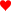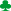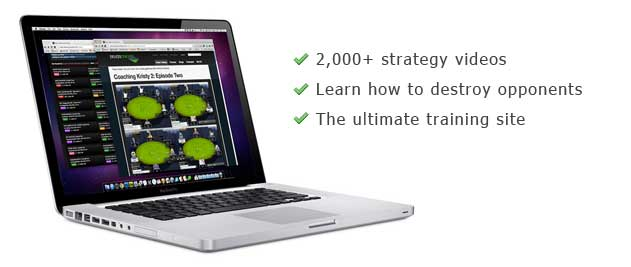# Poker Odds Conversion Table - Ratio & Percentage

Odds Charts: Ratio Chart : Percentage Chart : Conversion Chart

Percentage odds and ratio odds are thrown around and interchanged quite a lot when it comes to working out pot odds, drawing odds and all that mathematical stuff. With all the swapping around, it can be hard to keep up at time. What is 25% in ratio odds again?

There are two tables below to help you convert ratios to percentages and vice versa.

• The first table shows the exact odds that you would use based on the number of outs you have.
• The second table shows slightly rougher odds that you would use when quickly working out pot odds.
• e.g. if a you have to call \$5 to win a \$20 pot, that's 4 to 1 (or 20% chance if you convert it).

## Outs percentage and ratio conversions.

Outs 1 Card To Come (flop) - Ratio 1 Card To Come (flop) - %
1 46.0 to 1 2.1%
2 22.5 to 1 4.3%
3 14.7 to 1 6.4%
4 (gutshot) 10.8 to 1 8.5%
5 8.4 to 1 10.6%
6 6.8 to 1 12.8%
7 5.7 to 1 14.9%
8 (straight) 4.9 to 1 17.0%
9 (flush) 4.2 to 1 19.1%
10 3.7 to 1 21.3%
11 3.3 to 1 23.4%
12 2.9 to 1 25.5%
13 2.6 to 1 27.7%
14 2.4 to 1 29.8%
15 (s + f) 2.1 to 1 31.9%
16 1.9 to 1 34.0%
17 1.8 to 1 36.2%
18 1.6 to 1 38.3%
19 1.5 to 1 40.4%
20 1.4 to 1 42.6%
21 1.2 to 1 44.7%
22 1.1 to 1 46.8%

## Simple percentage and ratio conversions.

Ratio Percentage - %
10 to 1 9%
9 to 1 10%
8 to 1 11%
7 to 1 13%
6 to 1 14%
5 to 1 17%
4 to 1 20%
3 to 1 25%
2.5 to 1 29%
2 to 1 33%
1.5 to 1 40%
1 to 1 50%

Instead of referring to these tables all the time, try the handy hoRatio odds converter program.

## Outs odds table key.

• Gutshot - A straight draw with only one card able to complete it. e.g. 6-8 on a 5-9-Q board (only a 7 completes).
• Straight - A standard open-ended straight draw with more outs. e.g. 6-8 on a 5-7-Q board (4 and 9 complete).
• Flush - A hand where another card of the same suit is needed to complete the draw.
• s + f - Both an open ended straight draw and flush combined. e.g. 68on a 57Qboard.

## How to use the poker odds conversion charts.

The first chart is useful for comparing the ratio and percentage odds based on the number of outs you have. So you can easily see that a flush draw with 9 outs has odds to complete of 4.2 to 1 and 19.1%.

The second chart is useful for comparing and converting pot odds that you would be quickly working out on the spot. For example, a \$10 call to win a \$50 pot gives ratio pot odds of 5 to 1. As a percentage we can see that is roughly 17%.

As already mentioned, there is a free odds converter program that will convert any percentage or ratio odds in to the other format. It's called hoRatio and you can find it in the poker software section. You may find this more useful than referring to conversion tables.

### How to turn a ratio in to a percentage.

Add the two numbers in the ratio together. Then divide 100 by that number.

So for example, if you have a flush draw on the turn, the odds of completing your draw are 4.1 to 1 (let's call it 4 to 1).

• 4 to 1 odds, so 4 + 1 = 5.
• 100 / 5 = 20%.

So if you have 4 to 1 odds, you have a 20% chance of completing your draw. Easy.

Note: Your ratio must be in the format of "X to 1" first before this method will work. So for example, you would need to turn 7 to 2 in to 3.5 to 1 first. Most ratios in poker are in the X to 1 format anyway.

### How to turn a percentage in to a ratio.

Divide 100 by the percentage. Then take 1 away from that number and you will have x to 1.

So for example, if you have a flush draw on the turn, the percentage chance of completing your draw is 19.6% (let's call it 20%).

• 100 / 20 = 5.
• 5 - 1 = 4.
• So the ratio is 4 to 1.

It is a good idea to round the percentages to a number that you can easily divide in to 100 to help keep the working out as simple as possible.

Go back to the poker odds charts.

Have You Not Heard Of
Deuces Cracked?“I quit my job and started playing poker back in February of 08',
after a month of losing over 50BI at micro stakes, I joined DC. I've
been living off poker ever since; if anything in this world could help
you become a winning player, this is it.”
- Malefiicus, DC Member

# Subscribe to thepokerbank

I'll send you an email if I add something new and interesting to the website.

Don't worry, it doesn't happen very often.